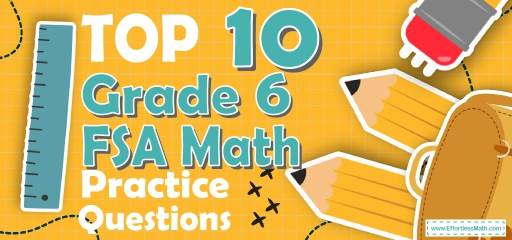# Top 10 6th Grade FSA Math Practice QuestionsAfter a thorough study of the 6th Grade FSA Math test resources, your students will need skill-building practice questions to review and practice all the test topics. The practice questions we have prepared in this article include the most common 6th Grade FSA Math practice questions that are most likely in the math section of the test. The answers to the questions are also well-explained so that the students can find out how they can solve a question. So, use our practice questions to help your students pass the 6th Grade FSA Math test easily.

Make sure to follow some of the related links at the bottom of this post to get a better idea of what kind of mathematics questions students need to practice.

The Absolute Best Book to Ace the FSA Math Test

## 6th Grade FSA Math Practice Questions

1- Which expression is equivalent to $$5(12x-16)$$?

A. $$-20$$

B. $$-20x$$

C. $$60x – 16$$

D. $$60x – 80$$

2- To produce a special concrete, for every 13 kg of cement, 3 liters of water is required. Which of the following ratios is the same as the ratio of cement to liters of water?

A. 91: 21

B. 14: 4

C. 39: 6

D. 9: 39

3- What is the value of $$x$$ in the following equation: $$-60=115-x$$

A. 175

B. $$-175$$

C. 55

D. $$-55$$

4- Which of the following graphs represents the following inequality?
$$-8≤5x-8≤2$$

A.

B.

C.

D.

5- The ratio of boys to girls in a school is 4:5. If there are 765 students in the school, how many boys are in the school?

A. 612

B. 510

C. 425

D. 340

6- Martin earns $20 an hour. Which of the following inequalities represents the amount of time Martin needs to work per day to earn at least$100 per day?

A. $$20t≥100$$

B. $$20t≤100$$

C. $$20+t≥100$$

D. $$20+t≤100$$

7- $$(55+5)÷12$$ is equivalent to …

A. $$60÷3.4$$

B. $$\frac{55}{12}+5$$

C. $$(2×2×3×5)÷(3×4)$$

D. $$(2×2×3×5)÷3+4$$

8- What is the value of the expression $$6(2x-3y)+(3-2x)^2$$, when $$x=2$$ and $$y=-1$$?

A. $$-23$$

B. 41

C. 43

D. 49

9- Round $$\frac{215}{7}$$ to the nearest tenth.

A. 31

B. 30.8

C. 30.7

D. 30

10- A chemical solution contains $$6\%$$ alcohol. If there is 45 ml of alcohol, what is the volume of the solution?

A. 270 ml

B. 420 ml

C. 750 ml

D. 1,200 ml

1- D
$$5(12x-16)=(5×12x)-(5×16)=(5×12)x-(5×16)=60x-80$$

2- A
$$91 : 21 = 13 : 3$$
$$13×7=91$$
And $$3×7=21$$

3- A
$$-60 = 115 – x$$
First, subtract 115 from both sides of the equation. Then:
$$-60 – 115=115 – 115- x→-175 = – x$$
Multiply both sides by $$(-1)$$:
$$→x = 175$$

4- B
$$-8≤5x-8<2 →$$ (add 8 all sides)$$-8+8≤5x-8+8<2+8 →0≤5x<10→$$ (divide all sides by 5) $$0≤x<2$$

5- D
The ratio of boys to girls is 4:5. Therefore, there are 4 boys out of 9 students. To find the answer, first, divide the total number of students by 9, then multiply the result by 4.
$$765 ÷ 9 = 85 ⇒ 85 × 4 = 340$$

6- A
For one hour he earns $20, then for t hours he earns$20t. If he wants to earn at least $100, therefore, the number of working hours multiplied by 20 must be equal to 100 or more than 100. $$20t≥100$$ 7- C $$(55+5)÷(12)=(60)÷(12)$$ The prime factorization of 60 is: $$2×2×3×5$$ The prime factorization of 12 is: $$3×4$$ Therefore: $$(60)÷(12)=(2×2×3×5)÷(3×4)$$ 8- C Plug in the value of $$x$$ and $$y$$ and use order of operations rule. $$x=2$$ and $$y=-1$$ $$6(2x-3y)+(3-2x)^2=6(2(2)-3(-1))+(3-2(2))^2=6(4+3)+(-1)^2 = 42+1=43$$ 9- C $$\frac{215}{7} ≅ 30.71 ≅ 30.7$$ 10- C $$6\%$$ of the volume of the solution is alcohol. Let $$x$$ be the volume of the solution. Then: $$6\% \space of \space x = 45 \space ml ⇒ 0.06 x = 45 ⇒ x = 45 ÷ 0.06 = 750$$ Looking for the best resource to help you succeed on the Grade 6 FSA Math test? The Most Comprehensive Review for 6th-Grade Students ## Related to This Article ### More math articles ### What people say about "Top 10 6th Grade FSA Math Practice Questions - Effortless Math: We Help Students Learn to LOVE Mathematics"? No one replied yet. X 51% OFF Limited time only! Save Over 51% SAVE$15

It was $29.99 now it is$14.99